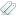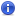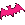Modul: export constant WerteTags: Similar Threads

Articles: hide open all | hide show old branches• User since
2011-03-20
815 articles
BenutzerInHallo!

Ist dieser Code in Ordnung? Das ist mein Versuch Term::Choose::Constants in Perl 6 umzuwandeln.

Code (perl): (dl )
```1
2
3
4
5
6
7
8
9
10
11
12
13
14
15
16
17
18
19
20
21
22
23
24
25
26
27
28
29
30
31
32
33
34
35
36
37
38
39
40
41
42
43
44
45
46
47
48
49
50
51
52
53
```
```use v6;

unit module Term::Choose::Constants;

constant ROW is export(:choose) = 0;
constant COL is export(:choose) = 1;
constant MIN is export(:choose) = 0;
constant MAX is export(:choose) = 1;

constant LF                     is export(:choose) = "\n";
constant CR                     is export(:choose) = "\r";

constant BEEP                   is export(:choose) = "\a";
constant CLEAR_SCREEN           is export(:linux)  = "\e[H\e[J";
constant CLEAR_TO_END_OF_SCREEN is export(:linux)  = "\e[0J";
constant RESET                  is export(:linux)  = "\e[0m";
constant BOLD_UNDERLINE         is export(:linux)  = "\e[1m\e[4m";
constant REVERSE                is export(:linux)  = "\e[7m";

constant HIDE_CURSOR            is export(:linux)  = "\e[?25l";
constant SHOW_CURSOR            is export(:linux)  = "\e[?25h";
constant WIDTH_CURSOR           is export(:choose) = 1;

constant GET_CURSOR_POSITION           is export(:linux) = "\e[6n";

constant SET_ANY_EVENT_MOUSE_1003      is export(:linux) = "\e[?1003h";
constant SET_EXT_MODE_MOUSE_1005       is export(:linux) = "\e[?1005h";
constant SET_SGR_EXT_MODE_MOUSE_1006   is export(:linux) = "\e[?1006h";
constant UNSET_ANY_EVENT_MOUSE_1003    is export(:linux) = "\e[?1003l";
constant UNSET_EXT_MODE_MOUSE_1005     is export(:linux) = "\e[?1005l";
constant UNSET_SGR_EXT_MODE_MOUSE_1006 is export(:linux) = "\e[?1006l";

constant MAX_ROW_MOUSE_1003            is export(:choose) = 223;
constant MAX_COL_MOUSE_1003            is export(:choose) = 223;

constant MOUSE_WHEELED                 is export(:win32) = 0x0004;

constant LEFTMOST_BUTTON_PRESSED       is export(:win32) = 0x0001;
constant RIGHTMOST_BUTTON_PRESSED      is export(:win32) = 0x0002;
constant FROM_LEFT_2ND_BUTTON_PRESSED  is export(:win32) = 0x0004;

constant NEXT_get_key  is export(:choose, :linux, :win32) = -1;

constant CONTROL_SPACE is export(:choose,         :win32) = 0x00;
constant CONTROL_A     is export(:choose                ) = 0x01;
# ...
# ...
constant VK_INSERT     is export(         :linux, :win32) = 45,   # unused
constant VK_DELETE     is export(         :linux, :win32) = 46,   # unused```

View all threads created 2015-08-11 12:50.